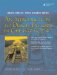# Constructors

A constructor, sometimes abbreviated as ctor, is a special member function that controls the process of object initialization. Each constructor must have the same name as its class. Constructors do not return anything and do not have return types.

There is a special syntax for constructor definitions:

```

ClassName::ClassName( parameter_list )
:init-list <-- 1
{
constructor body
}

```

(1)optional

Between the closing parenthesis of the parameter list and the opening brace of a function body, an optional member initialization list can be given. A member initialization list begins with a colon (:) and is followed by a comma-separated list of member initializers, each of the form

```member_name(initializing_expression)
```

If (and only if) no constructor is specified in a class definition, the compiler will supply one that looks like this:

```ClassName::ClassName()
{ }
```

A constructor that can be called with no arguments has the name default constructor. We say that a default constructor gives default initialization to an object of its class. Any data member that is not explicitly initialized in the member initialization list of a constructor is given default initialization.

Classes can have several constructors, each of which initializes in a different (and presumably useful) way. Example 2.7 has three constructors.

Example 2.7. src/ctor/complex.h

 ```class Complex { public: Complex(double realPart, double imPart); Complex(double realPart); Complex(); private: double m_R, m_I; }; ```

Example 2.8 shows the implementation with some client code.

Example 2.8. src/ctor/complex.cpp

 ```#include "complex.h" #include using namespace std; Complex::Complex(double realPart, double imPart) : m_R(realPart), m_I(imPart) <-- 1 { cout << "complex(" << m_R << "," << m_I << ")" << endl; } Complex::Complex(double realPart) { Complex(realPart, 0); <-- 2 } Complex::Complex() : m_R(0.0), m_I(0.0) { } int main() { Complex C1; Complex C2(3.14); Complex C3(6.2, 10.23); } ```   (1)member initialization list (2)Call one constructor from another, java-style.

The default constructor for this class gives default initialization to the two data members of the object C1. That initialization is the same kind that would be given to a pair of variables of type double in the following code fragment:

```double x, y;
cout << x << '	' << y << endl;
```

What would you expect to be the output of that code?

### SubobjectsAn Introduction to Design Patterns in C++ with Qt 4
ISBN: 0131879057
EAN: 2147483647
Year: 2004
Pages: 268